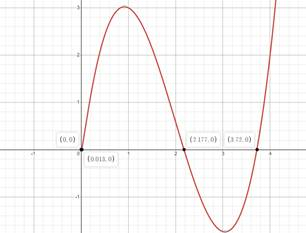# Two more solution of given equation except the interval of [ 1 , 6 ] .### Precalculus: Mathematics for Calcu...

6th Edition
Stewart + 5 others
Publisher: Cengage Learning
ISBN: 9780840068071### Precalculus: Mathematics for Calcu...

6th Edition
Stewart + 5 others
Publisher: Cengage Learning
ISBN: 9780840068071

#### Solutions

Chapter 1.9, Problem 71E
To determine

## Two more solution of given equation except the interval of [1,6] .

Expert Solution

x=0,0.013

### Explanation of Solution

Given information:

x36x2+9x=x

Formula used:

Solving by making graph.

Graphically:

Making Graph of x36x2+9x=xThe graph of given equation intersect to x-axis on (0,0),(0.013,0),(2.18,0),(3.72,0) . Our required solution are the ones that don’t lies in [1,6] , So,

x=0,0.013

### Have a homework question?

Subscribe to bartleby learn! Ask subject matter experts 30 homework questions each month. Plus, you’ll have access to millions of step-by-step textbook answers!